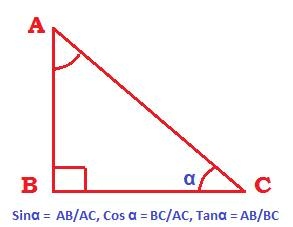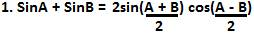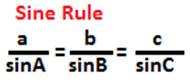## Trig-identities

1. Trig Identities on the six trigonometric ratios:

(Note: trig is an abbreviation for trigonometric. We will use trig for the word trigonometric to avoid repetition and therefore boredom.)1. Sinα = opposite side/hypotenuse = AB/AC

2. Cosα = adjacent side/hypotenuse = BC/AC

3. Tanα = opposite side/adjacent side = AB/BC

2. The reciprocal Trig identities

1. cosecα = 1/sinα

2. secα = 1/cosα

3. cotα = 1/tanα

3. The three Pythagorean trig identities

1. Sin2α + Cos2α = 1

2. Sec2α – tan2α = 1,  Sec2α =  1+ tan2α

3. Cosec2α – cotan2α = 1, Cosec2α = 1+ cotan2α

4. The quotient Trig identities:

1. tan α = sin α /cos α

2. cot α = cos α /sin α

5. Trig identities of negative angles:

1. Sin (– α) = –sin α

2. cos (– α) = cos α

3. tan (– α) = – tanα

4. cot (– α) = – cotα

5. Sec (– α) = Sec α

6. cosec (– α) = – cosec α

6. Trig identities of compound angles

1. sin (A + B) = sinAcosB + cosAsinB

2. sin (A – B) = sinAcosB – cosAsinB

3. cos (A + B) = cosAcosB – sinAsinB

4. cos (A – B) = cosAcosB + sinAsinB

5. Tan(A + B) = (sinAcosB + cosAsinB)/(cosAcosB – sinAsinB)

6. Tan(A – B) = (sinAcosB – cosAsinB)/(cosAcosB + sinAsinB)

7. Trig identities on products of compound angles

1. sin(A + B)sin(A – B) = sin2A – sin2B Or cos2B – cos2A

2. cos (A + B)cos(A – B) = cos2A – sin2B Or cos2B – sin2A

3. tan (A + B)tan(A – B) = (tan2A – tan2B)/ (1 – tan2Atan2B)

4. tan(A + B + C) = (tan A + tan B + tan C – tanA tanB tanC)/ (1– tanAtanB – tanB tanC –tanC tanA)

8. Trig identities on conversion of sum of angles to product of angles

1. sin (A + B) + sin ( A – B) = 2sinAcosB

2. sin (A + B) – sin (A – B) = 2 cosAsinB

3. cos ( A + B ) + cos (A – B) = 2cosAcosB

4. cos ( A – B ) – cos (A + B) = 2sinAsinB

9. Trig identities on sums of angles.10. trig identities on double angles:

1. Sin2A = 2sinAcosA Or 2tanA/ (1 + tan2A)

2. cos2A = 2cos2A – 1 Or 1 – 2sin2A Or (1 – tan2A)/(1 + tan2A)

3. tan2A = 2tanA/(1 – tan2A)

11. Trig identities on triple angles

1. Sin3A = 3sinA – 4sin3A

2. cos3A = 4cos3A – 3cosA

3. tan3A = (3tanA – tan3A)/(1 – 3tan2A)

12. Trig identities on sub-multiple angles

1. sin2A + sin2B + sin2C = 4sinAsinBsinC Or -1 – 4cosAcosBcosC

2. cos2A + cos2B + cos2C = 1 – 4 sinAsinBsinC

2. tan2A + tan2B + tan2C = tan2C tan2B tan2C

13. The law of sines Or the sine rule:14. the law of cosines or the cosine rule

a2 = b2 + c2 – 2bc cosA

b2 = a2 + c2 – 2ac cosB

c2 = b2 + a2 – 2abcosC

15. the projection formula

a = bcosC + ccosB

b = acosC + ccosA

c = bcosA + acosB

Algebraic identities.

Trig formulas.

Trig functions.

Trig equations.